## Heap-Tree

 Question 1

The number of possible min-heaps containing each value from {1, 2, 3, 4, 5, 6, 7} exactly once is ___________.

 A 80 B 81 C 82 D 83
Data-Structures       Heap-Tree       GATE 2018       Video-Explanation
Question 1 Explanation:
--> We have 7 distinct integers {1,2,3,4,5,6,7} and sort it
--> After sorting, pick the minimum element and make it the root of the min heap.
--> So, there is only 1 way to make the root of the min heap.
--> Now we are left with 6 elements.
--> Total ways to design a min heap from 6 elements = C(6,3) ∗ 2! ∗ C(3,3) ∗ 2! = 80

Note:
C(6,3)∗2! : Pick up any 3 elements for the left subtree and each left subtree combination can be permuted in 2! ways by interchanging the children and similarly, for right subtree .
 Question 2

A complete binary min-heap is made by including each integer in [1, 1023] exactly once. The depth of a node in the heap is the length of the path from the root of the heap to that node. Thus, the root is at depth 0. The maximum depth at which integer 9 can appear is _________.

 A 8 B 9 C 10 D 11
Data-Structures       Heap-Tree       GATE 2016 [Set-2]       Video-Explanation
Question 2 Explanation:This is not possible because it violates a property of complete binary tree.
We have total [0, 1023] elements. It means that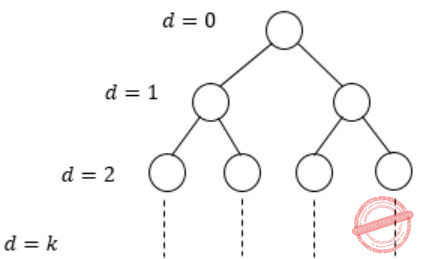∴ 20 + 21 + 22 + ⋯ + 2k = 1024
Add if 1(2(k+1)-1)/(2-1) [using formula for sum of k terms k+1 in G.P]
= 2(k+1) - 1 = 1024 - 1 = 1023
∴ The level ‘9’ at the depth of 8.
Actually we have 1023 elements, we can achieve a complete binary min heap of depth 9, which would cover all 1023 elements, but the max depth of node 9 can be only be 8.
 Question 3

Consider a complete binary tree where the left and the right subtrees of the root are max-heaps. The lower bound for the number of operations to convert the tree to a heap is

 A Ω(logn) B Ω(n) C Ω(nlog n) D Ω(n2)
Data-Structures       Heap-Tree       GATE 2015 [Set-2]
Question 3 Explanation:
Since left and right subtree is already a heap. So we can apply Heapify (node) which take log n time.
 Question 4

Consider the following array of elements. 〈89, 19, 50, 17, 12, 15, 2, 5, 7, 11, 6, 9, 100〉. The minimum number of interchanges needed to convert it into a max-heap is

 A 4 B 5 C 2 D 3
Data-Structures       Heap-Tree       GATE 2015 [Set-3]
Question 4 Explanation:
Let's first draw heap from the given array,→ Swap 100 & 15→ Swap 100 & 50→ Swap 89 & 100∴ Total 3 interchanges are needed.
 Question 5

Consider a binary max-heap implemented using an array.

Which one of the following array represents a binary max-heap?

 A {25,12,16,13,10,8,14} B {25,14,13,16,10,8,12} C {25,14,16,13,10,8,12} D {25,14,12,13,10,8,16}
Data-Structures       Heap-Tree       GATE 2009
Question 5 Explanation:
Option-A:Violating a max heap property.
Option-B:Violating a max heap property.
Option-C: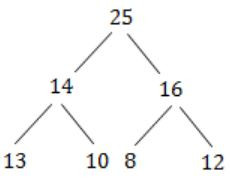Question 6

Consider a binary max-heap implemented using an array.

What is the content of the array after two delete operations on the correct answer to the previous question?

 A {14,13,12,10,8} B {14,12,13,8,10} C {14,13,8,12,10} D {14,13,12,8,10}
Data-Structures       Heap-Tree       GATE 2009
Question 6 Explanation:
Actual Graph: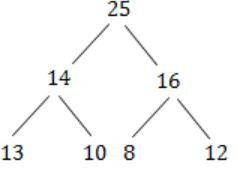Step 1: Delete 25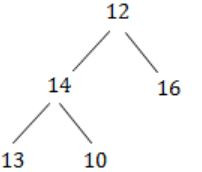Step 2:Step 3: Delete 16Step 4:Step 5: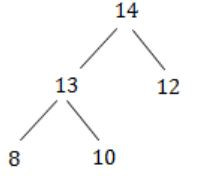∴ Binary array elements: 14, 13, 12, 8, 10.
 Question 7

We have a binary heap on n elements and wish to insert n more elements (not necessarily one after another) into this heap. The total time required for this is

 A θ(log n) B θ(n) C θ(nlog n) D θ(n2)
Data-Structures       Heap-Tree       GATE 2008
Question 7 Explanation:
Inserting an element into binary(either max or min) heap takes O(logn) for all cases, but in question they clearly mentioned that n elements and inserting one by one n elements, so it takes 2n time. So, O(n).
Note: We can also insert all the elements once, there will be no difference on time complexity.
 Question 8

Consider the process of inserting an element into a Max Heap, where the Max Heap is represented by an array. Suppose we perform a binary search on the path from the new leaf to the root to find the position for the newly inserted element, the number of comparisons performed is:

 A θ(log2n) B θ(log2log2n) C θ(n) D θ(nlog2n)
Data-Structures       Heap-Tree       GATE 2007
Question 8 Explanation:
Max heap is the complete binary tree that means each node has either zero children or two children except last level. So in worst case insertion of element is at last level. So, number of comparisons required at each level starting from root is equal to 1+2+4+8+16+---- this series is equal to "logn". All the elements are sorted, the binary search which will result in O(loglogn) number of comparisons.
 Question 9

In a binary max heap containing n numbers, the smallest element can be found in time

 A O(n) B O(log n) C O(log log n) D O(1)
Data-Structures       Heap-Tree       GATE 2006
Question 9 Explanation:
In a MAX heap the smallest values of the heap will always be present on the last level of heap and time complexity of reaching the last level of heap is O(n).
We have to search all the elements to reach the smallest element and heap using linear search.
To traverse all elements using linear search will take O(n) time.
 Question 10

A 3-ary max heap is like a binary max heap, but instead of 2 children, nodes have 3 children. A 3-ary heap can be represented by an array as follows: The root is stored in the first location, a, nodes in the next level, from left to right, is stored from a to a. The nodes from the second level of the tree from left to right are stored from a location onward. An item x can be inserted into a 3-ary heap containing n items by placing x in the location a[n] and pushing it up the tree to satisfy the heap property.

Which one of the following is a valid sequence of elements in an array representing 3-ary max heap?

 A 1, 3, 5, 6, 8, 9 B 9, 6, 3, 1, 8, 5 C 9, 3, 6, 8, 5, 1 D 9, 5, 6, 8, 3, 1
Data-Structures       Heap-Tree       GATE 2006
Question 10 Explanation:Question 11

A 3-ary max heap is like a binary max heap, but instead of 2 children, nodes have 3 children. A 3-ary heap can be represented by an array as follows: The root is stored in the first location, a, nodes in the next level, from left to right, is stored from a to a. The nodes from the second level of the tree from left to right are stored from a location onward. An item x can be inserted into a 3-ary heap containing n items by placing x in the location a[n] and pushing it up the tree to satisfy the heap property.

Suppose the elements 7, 2, 10 and 4 are inserted, in that order, into the valid 3-ary max heap found in the above question, Q.76. Which one of the following is the sequence of items in the array representing the resultant heap?

 A 10, 7, 9, 8, 3, 1, 5, 2, 6, 4 B 10, 9, 8, 7, 6, 5, 4, 3, 2, 1 C 10, 9, 4, 5, 7, 6, 8, 2, 1, 3 D 10, 8, 6, 9, 7, 2, 3, 4, 1, 5
Data-Structures       Heap-Tree       GATE 2006
Question 11 Explanation: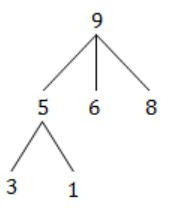Question 12

The elements 32, 15, 20, 30, 12, 25, 16 are inserted one by one in the given order into a Max Heap. The resultant Max Heap is

 ABC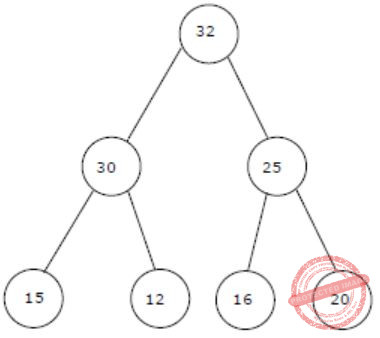DData-Structures       Heap-Tree       GATE 2004
Question 12 Explanation:
32, 15, 20, 30, 12, 25, 16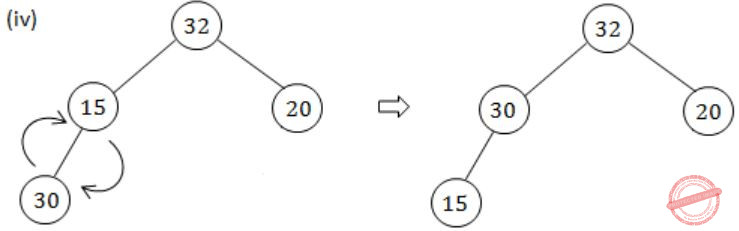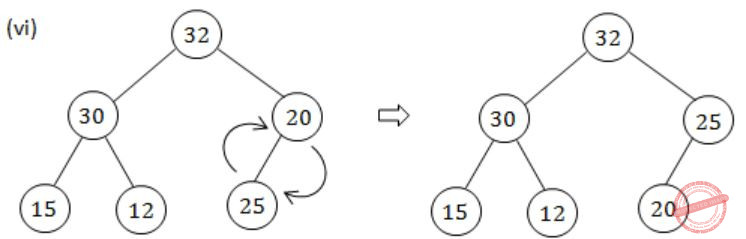Question 13

In a heap with n elements with the smallest element at the root, the 7th smallest element can be found in time

 A Θ(n log n) B Θ(n) C Θ(log n) D Θ(1)
Data-Structures       Heap-Tree       GATE 2003
Question 13 Explanation:
The 7th smallest elements can be present in any of 7 levels. Then total possible elements can be present is seven levels is
1 + 2 + 4 + 6 + 8 + 16 + 32
Which is constant then we can find the 7th smallest element in Θ(1) time.
 Question 14

Consider any array representation of an n element binary heap where the elements are stored from index 1 to index n of the array. For the element stored at index i of the array (i≤n), the index of the parent is

 A i-1 B ⌊i/2⌋ C ⌈i/2⌉ D (i+1)/2
Data-Structures       Heap-Tree       GATE 2001
Question 14 Explanation:
Parent Node is at index: ⌊i/2⌋
Left Child is at index: 2i
Right child is at index: 2*i+1
 Question 15

The minimum number of interchanges needed to convert the array

` 89, 19, 40, 17, 12, 10, 2, 5, 7, 11, 6, 9, 70  `

into a heap with the maximum element at the root is

 A 0 B 1 C 2 D 3
Data-Structures       Heap-Tree       GATE 1996
Question 15 Explanation:
Lets draw first heap from given sequence,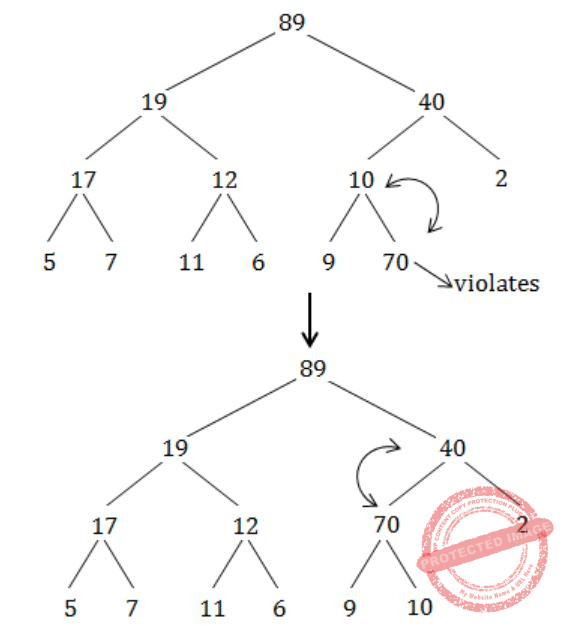Question 16

Consider the array representation of a binary min-heap containing 1023 elements. The minimum number of comparisons required to find the maximum in the heap is _______.

 A 511
Data-Structures       Heap-Tree       GATE 2020
Question 16 Explanation:
The binary heap contains 1023 elements. When it performs minimum comparisons it will take Ceil(n/2)
n=1023
= Ceil(1023/2)
= 512
So, the maximum element is also part of n/2.
So, we have to subtract from the total elements
= 512-1
= 511
 Question 17

Which of the following sequences of array elements forms a heap?

 A {23, 17, 14, 6, 13, 10, 1, 12, 7, 5} B {23, 17, 14, 6, 13, 10, 1, 5, 7, 12} C {23, 17, 14, 7, 13, 10, 1, 5, 6, 12} D {23, 17, 14, 7, 13, 10, 1, 12, 5, 7}
Data-Structures       Heap-Tree       GATE 2006-IT
Question 17 Explanation:In this every children and parent satisfies Max heap properties.
 Question 18

Consider the array A = 〈4, 1, 3, 2, 16, 9, 10, 14, 8, 7〉. After building heap from the array A, the depth of the heap and the right child of max-heap are and respectively. (Root is at level 0).

 A 3, 14 B 3, 10 C 4, 14 D 4, 10
Data-Structures       Heap-Tree       UGC-NET CS 2018 JUNE Paper-2
Question 18 Explanation:
A heap is a MAX-Heap if all the root nodes (parent nodes) have maximum value.
Step 1: Since a heap is a almost complete binary tree so build a heap first.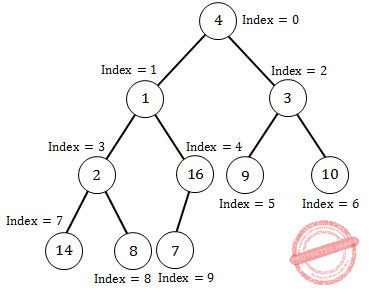Step 2: Since the above heap is not a max heap so to convert a heap into max-heap start applying max-heapify operation from the largest index parent node (node having 1 or more children).The above Heap is the max-heap where each root node have maximum value.
Now depth of the Max-heap is 3 and right child of the Root node is 10.
 Question 19
We have a binary heap on n elements and wish to insert n more elements (not necessarily one after another) into this heap. Total time required for this is
 A O(logn) B O(n) C O(nlogn) D O(n​ 2​ )
Data-Structures       Heap-Tree       Nielit Scientist-C 2016 march
Question 19 Explanation:
Possibility-1: Inserting an element into binary(either max or min) heap takes O(logn) for all cases, but in question they clearly mentioned that n elements. O(n) complexity can be to take the ‘n’ elements of the heap and other ‘n’ elements together and construct heap So, O(n).
Possibility-2: If you inserted one after the other then time complexity will be O(nlogn). For one insertion it will take O(logn) because need to apply maxHeapify. For n elements it will be O(nlogn)
Note: We can also insert all the elements once, there will be no difference on time complexity.
But according GATE solution they given possibility-1.
 Question 20
Consider the process of inserting an element into a Max Heap, where the Max Heap is represented by an array. Suppose we perform a binary search on the position for the newly inserted element, the number of comparisons performed is
 A O(log​ 2​ n) B O(nlog​ 2​ n) C O(n)
Data-Structures       Heap-Tree       Nielit Scientist-C 2016 march
Question 20 Explanation:
Max heap is the complete binary tree that means each node has either zero children or two children except last level. So in worst case insertion of element is at last level. So, number of comparisons required at each level starting from root is equal to 1+2+4+8+16+---- this series is equal to "logn".
Note: If you apply linear search it will take O(logn) and binary search it will take O(loglogn). If you want to insert an element into already created heap is O(logn) because you need to apply maxheapify.
 Question 21

The elements 42, 25, 30, 40, 22, 35, 26 are inserted one by one in the given order into a max-heap. The resultant max-heap is stored in an array implementation as

 A <42, 35, 40, 22, 25, 26, 30> B <42, 35, 40, 22, 25, 30, 26> C <42, 40, 35, 25, 22, 26, 30> D <42, 40, 35, 25, 22, 30, 26>
Data-Structures       Heap-Tree       UGC-NET CS 2018 DEC Paper-2
Question 21 Explanation:
After inserting each element we will apply MAX-Heapify operation to get the MAX-Heap.
The resultant MAX_Heap will look like<42, 40, 35, 25, 22, 30, 26>
 Question 22
In a binary max heap containing n numbers, the smallest element can be found in time
 A O(n) B O(logn) C O(loglogn) D O(A)
Data-Structures       Heap-Tree       NieLit STA 2016 March 2016
Question 22 Explanation:
In Binary Max Heap, the smallest element will always be a leaf node either in left subtree or right subtree. So you have to traverse both left and right subtrees in each step to find the smallest element. So, it takes O(n) time.
 Question 23
Which languages necessarily need heap allocation in the run time environment?
 A Those that support recursion B Those that use dynamic scoping C Those that use global variables D Those that allow dynamic data structures
Data-Structures       Heap-Tree       ISRO DEC 2017 22- Soon
Question 23 Explanation:
→ Dynamic memory is allocated on the heap by the system.
→ So the languages which allow dynamic data structure require heap allocation at runtime.
 Question 24
Which of the following is a valid heap ?A A B B C C D D
Data-Structures       Heap-Tree       UGC NET CS 2017 Jan -paper-2
Question 24 Explanation:
Option A is violated max heap property because 8 is greater than his root.
Option B is satisfied max heap property.
Option C is violated max heap property because 7 is greater than his root.
Option D is violated max heap property because 9,10,8,7 elements are greater than his root.
 Question 25
The elements 42, 25, 30, 40, 22, 35, 26 are inserted one by one in the given order into a max-heap. The resultant max-heap is stored in an array implementation as
 A <42, 35, 40, 22, 25, 26, 30> B <42, 35, 40, 22, 25, 30, 26> C <42, 40, 35, 25, 22, 26, 30> D <42, 40, 35, 25, 22, 30, 26>
Data-Structures       Heap-Tree       UGC NET CS 2018-DEC Paper-2
Question 25 Explanation:
After inserting each element we will apply MAX-Heapify operation to get the MAX-Heap.
The resultant MAX_Heap will look like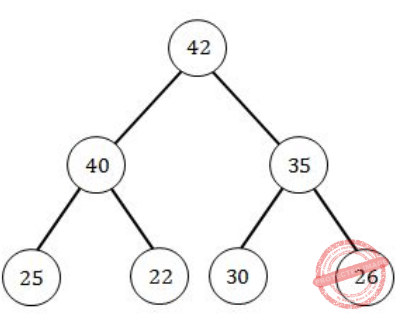< 4 2, 40, 35, 25, 22, 30, 26 >
 Question 26
Consider the array A=<4, 1, 3, 2, 16, 9, 10, 14, 8, 7>. After building heap from the array A, the depth of the heap and the right child of max-heap are__________ and __________ respectively. (Root is at level 0).
 A 3, 14 B 3, 10 C 4, 14 D 4, 10
Data-Structures       Heap-Tree       UGC NET CS 2018 JUNE Paper-2
Question 26 Explanation:
A heap is a MAX-Heap if all the root nodes(parent nodes) have maximum value.
Step 1: Since a heap is a almost complete binary tree so build a heap first.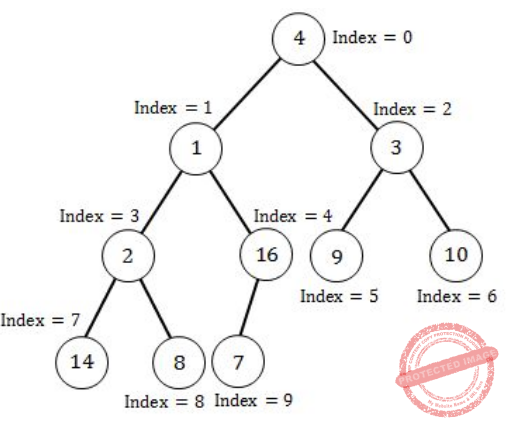Step 2: Since the above heap is not a max heap so to convert a heap into max-heap start applying max- heapify operation from the largest index parent node(node having 1 or more children).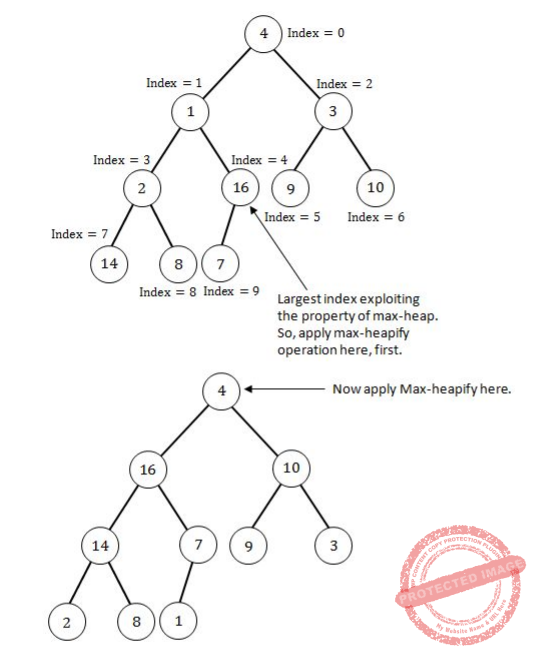The above Heap is the max-heap where each root node have maximum value. Now depth of the Max-heap is 3 and right child of the Root node is 10.
 Question 27
When the priority queue is represented by max heap, the insertion and deletion of an element can be performed in (queue containing n elements)
 A θ(n) and θ(1) respectively B θ(n) and θ(n) respectively C θ(1) and θ(1) respectively D None of the above
Data-Structures       Heap-Tree       UGC NET CS 2011 June-Paper-2
Question 27 Explanation:Question 28
The time complexity to build a heap with a list of n numbers is
 A O(log n) B O(n) C O(n logn) D O(n2)
Data-Structures       Heap-Tree       UGC NET CS 2013 June-paper-2
Question 28 Explanation:
Build-Max-Heap(A)
// Input: A: an (unsorted) array
// Output: A modified to represent a heap.
// Running Time: O(n) where n = length[A]
1 heap-size[A] ← length[A]
2 for i ← Floor(length[A]/2) downto 1
3 Max-Heapify(A, i)
The Build-Max-Heap function that follows, converts an array A which stores a complete binary tree with n nodes to a max-heap by repeatedly using Max-Heapify in a bottom up manner. It is based on the observation that the array elements indexed by floor(n/2) + 1, floor(n/2) + 2, ..., n are all leaves for the tree (assuming that indices start at 1), thus each is a one-element heap.
→ Build-Max-Heap runs Max-Heapify on each of the remaining tree nodes.
 Question 29
The time complexity to build a heap of n elements is
 A O(1) B O(lgn) C O(n) D O(nlgn)
Data-Structures       Heap-Tree       UGC NET CS 2010 Dec-Paper-2
Question 29 Explanation:
The time complexity to build a heap of n elements is O(n).
Build-Max-Heap(A)
// Input: A: an (unsorted) array
// Output: A modified to represent a heap.
// Running Time: O(n) where n = length[A]
1 heap-size[A] ← length[A]
2 for i ← Floor(length[A]/2) downto 1
3 Max-Heapify(A, i)
The Build-Max-Heap function that follows, converts an array A which stores a complete binary tree with n nodes to a max-heap by repeatedly using Max-Heapify in a bottom up manner. It is based on the observation that the array elements indexed by floor(n/2) + 1, floor(n/2) + 2, ..., n are all leaves for the tree (assuming that indices start at 1), thus each is a one-element heap.
→ Build-Max-Heap runs Max-Heapify on each of the remaining tree nodes.
 Question 30
In a heap, every element is __________ of all the elements in the subtree.
 A maximum B minimum C sum D product E None of the above
Data-Structures       Heap-Tree       UGC NET CS 2008 Dec-Paper-2
Question 30 Explanation:
Heap is complete binary tree. Sometimes we are calling as binary heap that may be either Max heap or Min heap.
In Max heap→ The root element is greater than of his all childs.
In Min heap→ Te root element is smaller than of his all childs.
 Question 31
When a user submits a query, a search engine does the following. For every webpage that has been visited by the search engine, it computes a score indicating how relevant that page is to the query. Finally, it reports the pages with the top k scores on the screen, for a number k specified by the user. A good data structure for accumulating the scores and ranking them is:
 A a queue B a heap C a stack D a binary search tree
Data-Structures       Heap-Tree       CHENNAI MATHEMATICAL INSTITUTE (M.Sc. / Ph.D. Programme in Computer Science)15 May 2013
Question 31 Explanation:
Let n be the number of pages visited by the search engine at the time a query is submitted. Assume that it takes constant time to compute the relevance score for each page w.r.t. a query. Then it takes O(n) time to compute the relevance scores, a further O(n) time to build a heap of n relevance scores, and O(k · log n) time for k delete-max operations to return the top k scores.
 Question 32
A priority queue is implemented as a max-heap. Initially, it has ve elements. The level-order traversal of the heap is as follows:  20, 18, 15, 13, 12  Two new elements ‘10’ and ‘17’are inserted in the heap in that order. The level-order traversal of the heap after the insertion of the element is:
 A 20, 18, 17, 15, 13, 12, 10 B 20, 18, 17, 12, 13, 10, 15 C 20, 18, 17, 10, 12, 13, 15 D 20, 18, 17, 13, 12, 10, 15
Data-Structures       Heap-Tree       UGC NET CS 2016 Aug- paper-3
Question 32 Explanation:Question 33
The number of nodes of height ‘h’ in any n-element heap is __________.
 A h B z​ h C ⌈n/(z​ h​ )⌉ D ⌈n/z​ h+1​ ) E None of the above
Data-Structures       Heap-Tree       UGC NET CS 2015 June Paper-3
Question 33 Explanation:Question 34
Construct a Max-heap dynamically to accomodate a stream of eight integers, 48,34,68,32,19,25,61,53 as a descending priority queue. The leaf node that is farthest from the root is ______
 A 19 B 68 C 48 D 32
Data-Structures       Heap-Tree       APPSC-2016-DL-CS
Question 34 Explanation:Question 35

A min-max heap is defined as follows: Each node at an even level in the tree is less than all of its descendants, while each node at an odd Level in the tree is greater than all of its descendents

If the values are inserted in the order (3,6,9,19,27,48,50,66) in the min-max heap, what value would be found at position 5 in min-max heap (root is counted as position 1)
 A 6 B 27 C 48 D 50 E None of the above
Data-Structures       Heap-Tree       HCU PHD CS MAY 2015
Question 35 Explanation: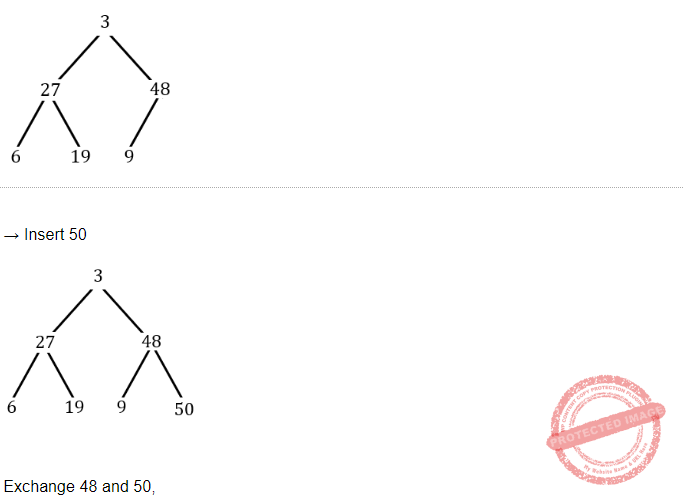Question 36
What is the time required to insert n more elements to a binary heap of n elements?
 A O(nlog n) B O(log n) C O(n^2) D O(n)
Data-Structures       Heap-Tree       HCU PHD CS MAY 2016
Question 36 Explanation:
To insert 1 element it requires O(logn) time.So to insert n elements it requires O(nlogn) time.
 Question 37
In a binary max heap containing n numbers, the smallest element can be found in_____ time.
 A O(n) B O(log2 n) C O(1) D O(log2 log2 n)
Data-Structures       Heap-Tree       UGC NET JRF November 2020 Paper-2
Question 37 Explanation:
In a MAX heap the smallest values of the heap will always be present on the last level of heap and time complexity of reaching the last level of heap is O(n).
We have to search all the elements to reach the smallest element and heap using linear search.
To traverse all elements using linear search will take O(n) time.
There are 37 questions to complete.